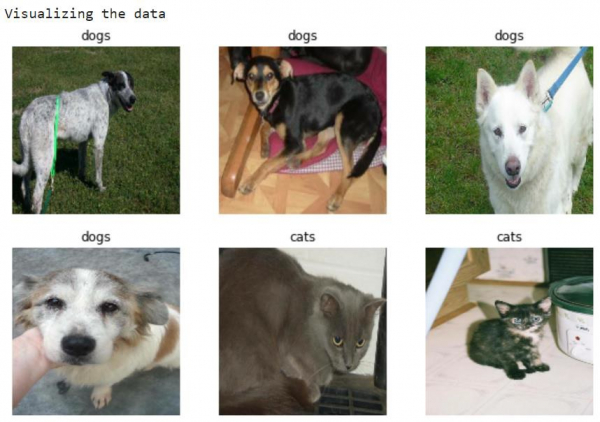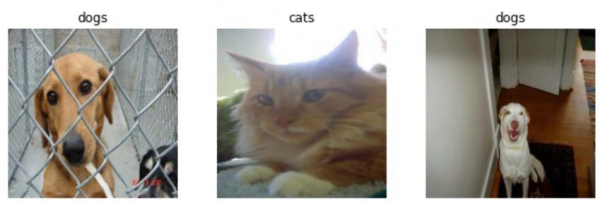# How can Tensorflow and pre-trained model after recompiling be used to visualize the data?

Tensorflow and the pre-trained model can be used to visualize the data using the ‘matplotlib’ library. The ‘imshow’ method is used to display the data on the console.

A neural network that contains at least one layer is known as a convolutional layer. We can use the Convolutional Neural Network to build learning model.

We will understand how to classify images of cats and dogs with the help of transfer learning from a pre-trained network. The intuition behind transfer learning for image classification is, if a model is trained on a large and general dataset, this model can be used to effectively serve as a generic model for the visual world. It would have learned the feature maps, which means the user won’t have to start from scratch by training a large model on a large dataset.

We are using the Google Colaboratory to run the below code. Google Colab or Colaboratory helps run Python code over the browser and requires zero configuration and free access to GPUs (Graphical Processing Units). Colaboratory has been built on top of Jupyter Notebook.

## Example

print("Visualizing the data")
plt.figure(figsize=(10, 10))
for i in range(9):
ax = plt.subplot(3, 3, i + 1)
plt.imshow(image_batch[i].astype("uint8"))
plt.title(class_names[predictions[i]])
plt.axis("off")

## Output## Explanation

• When a small dataset is used, the features can be learned by a model which is trained on a larger dataset in the same domain.

• This can be done by instantiating pre-trained model and adding a fully-connected classifier on top.

• The pre-trained model is "frozen" and the weights of the classifier are updated during training only.

• The convolutional base will extract all the features associated with each image.

• To improve performance, the top-level layers of the pre-trained models can be fine-tuned.

• This is done so that model learns high-level features specific to that dataset.

• This technique is recommended when training dataset is large and is similar to the original dataset which the pre-trained model was trained on.